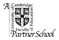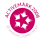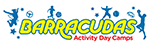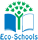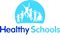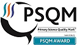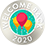# Maths

• Count and calculate in a range of practical contexts.
• Use and apply mathematics in everyday activities and across the curriculum.
• Repeat key concepts in many different practical ways to secure retention.
• Explore numbers and place value up to at least 100.
• Add and subtract using mental and formal written methods in practical contexts.
• Multiply and divide using mental and formal written methods in practical contexts.
• Explore the properties of shapes.
• Use language to describe position, direction and movement.
• Use and apply in practical contexts a range of measures, including time.
• Handle data in practical contexts.